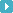## 数学乘法结合律和交换律专项练习题

2015-08-10 14:07:58 来源：x.dgqjj.com 浏览：15

1、填空题，在划线上填写数字或字母，使得等式两边成立。

①400×______×8 = 400×(15×8)

②(a×b)×c = a×(_____×_____)

③35×______ = 46×_______

④45×5×4 = 45×(______×_____)

⑤100×32×25 = (10×______)×(_____×_______)

⑥a×b = _____×_____

2、符合乘法交换律的画○,符合结合律的画△

①35×28=28×35 (     )

②32×25=8×(4×25)(    )

③25×15×4×2=(15×2)×(25×4)(     )

④ a×b×c=a×c×b(    )

3、算一算，想一想，你有什么发现？

(1)30×2×5=     30×(2×5)=       我发现：_______________________

(2)25 ×16 ×4=    (25 ×4)×16=   我发现：_______________________

3、简算

33×15×2          25×7×4×3      25×50×8       25×125×16

125×32×25        4×(25×9)       16×25×125     38×5×4

5×(19×2)         4×45×25        25×23×8       125×72

(25×125)×32      (30×25)×40     (15×25)×4      15×(25×4)

(6×12)×5         6×(12×5)       (13×5)×20      5×(13×20)

125×48            35×2×5          (60×25)×4      125×5×8

4、请用乘法交换律或乘法结合律解答以下应用题。

A、东莞东城某市场进了56箱鸡蛋，每箱25千克，每千克鸡蛋8元，这一批鸡蛋一共可卖多少钱？

B、在莞城中心小学举行的体操表演中，一共有关5个方阵参加表演，每个方阵有18行，每行都有20 名同学，一共有多少名同学？

C、 有8个书架，每个书架都有7层，每层可放125 本书，这些书架一共可放多少本书？相关文章发表评论最近更新点击排行